# Appliyng Gradient Descent to Train Neural Networks

in #bloglast year

This post, similar to the previous one, will focus on how a neural network can identify handwritten digits. This is a prevalent introductory problem to neural networks. You could call it the “Hello World!” problem for the field of neural networks.

This is the fifth post in a series on neural networks and deep learning. This series is my attempt to get more familiar with the topic and is heavily based on the book by Michael Nielsen.

The starting point of this post is the previous discussion on a gradient descent to find minima of functions of arbitrary degrees of freedom. Here we will use his technique to find the weights $k_w$ and biases $b_l$ which minimize the cost function of a neural net shown below: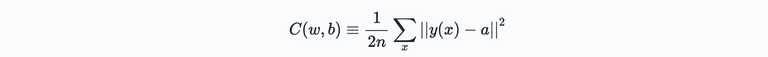To achieve this, lets replace the variables $v_j$ used previously by the weights and biases $w_k$ and $b_l$ and define the gradient of cost function $C$ as follows: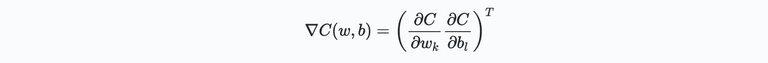Hence in this case the "position" defined by the weights an biases is modified such that the cost function is at it's minimum. The updates of the degrees of freedom of our function $C$ are now defined as:By iteratively Applying these update rules, we will likely find the minimum of the cost function and, by doing so, improving our neural network.

This approach seems straightforward. But, as always, the method comes with some challenges. Challenges which we will come across at a later stage. However, let's already discuss one major problem. For this, let's look at our cost function again: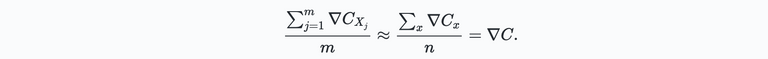This function is quadratic. It has the form $C=\frac{1}{n}\sum_x C_x$. That is, it's an average over costs $C_x \equiv \frac{||y(x) - a||^2}{2}$ for individual training examples. Moreover, to compute the gradients $nabla C_x$ for each training input $x$ and then average them: $nabla C_x = \frac{1}{n} \sum_x \nabla C_x$. Hence, when we have a huge number of training inputs, it can take a very Hence, when we have a very large number of training inputs, it can take a very long time, and learning will be slow

There are different techniques to speed up this process. One approach is stochastic gradient descent. The main idea is to compute the gradient $\nabla C$ using only a subset of randomly chosen training inputs. Hence only a reduced number of gradients $\nabla C_x$ need to be computed this way. Although this would not result in the exact gradient, it turns out that it works quite well in finding the minimum quickly, and thus learning speed is improved.

To define stochastic gradient descent more explicitly, we take $m$ randomly chosen training inputs and label them $X_1, X_2,...,X_m$. We refer to these as a mini-batch. Given that the batch size $m$ is big enough the approximated average $\nabla C_{X_j}$ will be approaching the overall average $\nabla C_{x}$, or: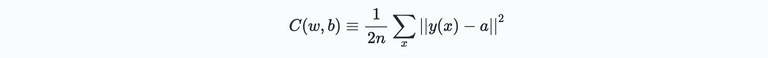Here, the second sum is over the complete set of training data with size $n$. To make the connection with the weights and biases, we apply this technique be simply choosing a random subset of the weights and biases and use this to train the network:Here, the sums are over all the training examples $X_j$ within the mini batch. Once the update is chosen, we select a new randomly chosen mini-batch and repeat to process to train those. Once all training inputs have been used, a so-called epoch of training is completed.

Note that the stochastic approach introduced above is not exact. It's an approximation of the result obtained from a complete set. However, it works generally well and reduces learning time significantly.

Ok, that's it for now! In the next post, we will be working on some actual code! Stay tuned...

#### Previous Articles on Neural Networks

Sort:

Congratulations @michelmake! You have completed the following achievement on the Hive blockchain and have been rewarded with new badge(s):You received more than 8000 upvotes.Your next target is to reach 9000 upvotes.

If you no longer want to receive notifications, reply to this comment with the word STOP

Check out the last post from @hivebuzz: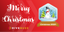Merry Christmas - Challenge Feedback - Win a 1000 HP delegation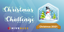Christmas Challenge - Offer a gift to to your friends
###### Support the HiveBuzz project. Vote for our proposal!

Ho Ho Ho! @michelmake, one of your Hive friends wishes you a Merry Christmas and asked us to give you a new badge!The HiveBuzz team wish you a Merry Christmas!May you have good health, abundance and everlasting joy in your life.Merry Christmas - Challenge Feedback - Win a 1000 HP delegation